# Algebra II : Adding and Subtracting Fractions

## Example Questions

### Example Question #69 : Fractions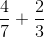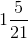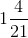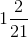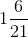Explanation:

When adding fractions, the first thing we need to do is get a common denominator.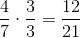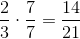Then when adding, only add the numbers on the top, while leaving the common number on the bottom the same. Once we get a final answer, simplify accordingly.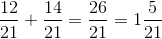### Example Question #71 : Fractions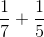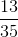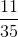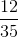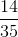Explanation:

When adding fractions, the first thing we need to do is get a common denominator.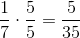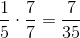Then when adding, only add the numbers on the top, while leaving the common number on the bottom the same. Once we get a final answer, simplify accordingly.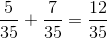### Example Question #72 : Fractions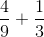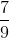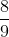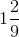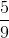Explanation:

When adding fractions, the first thing we need to do is get a common denominator.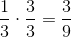Then when adding, only add the numbers on the top, while leaving the common number on the bottom the same. Once we get a final answer, simplify accordingly.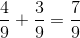### Example Question #73 : Fractions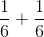Explanation:

When adding fractions, the first thing we need to do is get a common denominator, but for this question, we do not need to do this because their denominators are already the same. Then when adding, only add the numbers on the top, while leaving the common number on the bottom the same. Once we get a final answer, simplify accordingly.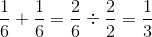### Example Question #74 : Fractions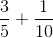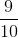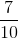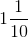Explanation:

When adding fractions, the first thing we need to do is get a common denominator.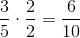Then when adding, only add the numbers on the top, while leaving the common number on the bottom the same. Once we get a final answer, simplify accordingly.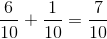### Example Question #75 : Fractions

Subtract the fractions: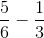Explanation:

When subtracting fractions, the first thing we need to do is get a common denominator.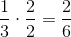Then when subtracting, only subtract the numbers on the top, while leaving the common number on the bottom the same. Once we get a final answer, simplify accordingly.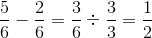### Example Question #76 : Fractions

Subtract the fractions: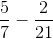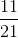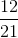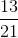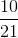Explanation:

When subtracting fractions, the first thing we need to do is get a common denominator.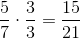Then when subtracting, only subtract the numbers on the top, while leaving the common number on the bottom the same. Once we get a final answer, simplify accordingly.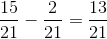### Example Question #77 : Fractions

Subtract the fractions: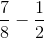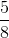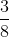Explanation:

When subtracting fractions, the first thing we need to do is get a common denominator.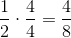Then when subtracting, only subtract the numbers on the top, while leaving the common number on the bottom the same. Once we get a final answer, simplify accordingly.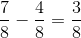### Example Question #78 : Fractions

Combine the fractions and leave answer as either whole number or fraction.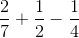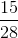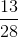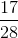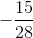Explanation:

First you must find the Least Common Denominator which is 28.

So you will multiply as follows to get the common denominator of 28: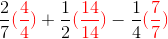Making it: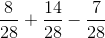### Example Question #79 : Fractions

If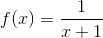and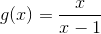, what is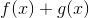?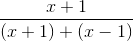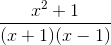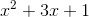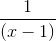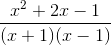Explanation:

Let's first write the equation out: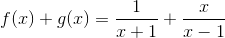In order to add these fractions, we need to have a common denominator.  To do this, we multiply the first term by the denominator of the second term, and vise versa: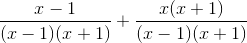Now we can add the numerators, while the denominators would stay the same: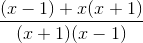We then simplify the numerator to get the final answer: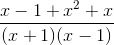### All Algebra II Resources